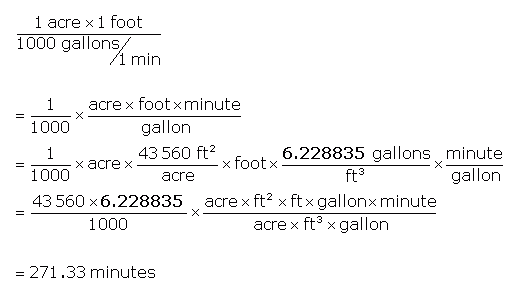SEARCH HOMEMath Central Quandaries & QueriesQuestion from Jeff: If you have one square acre of ground flooded with one foot of water and a pump that pumps 1000 gallons a minute how long would it take to drain the one acre of ground?Hi Jeff,

One acre is 43560 square feet so the volume of water is 435601 = 43560 cubic feet. One US gallon is 231 cubic inches which is 231/(121212) = 0.13368 cubic feet. Since you can pump at 1000 gallons per minute that is 133.68 cubic feet per minute. Hence to pump 43560 cubic feet will take

43560/133.68 = 325.85 minutes.

Since there are 60 minutes in an hour that is

325.85/60 = 5.43 hours.

Penny

Jeff,

Here's how I would work this out. First remember that time = volume / pumprate.Note that I used UK gallons here. If you are using US gallons, you need to use 7.480519 instead of 6.228835 because US gallons are smaller than UK gallons.

Stephen La Rocque.>Math Central is supported by the University of Regina and The Pacific Institute for the Mathematical Sciences.